# M&M Math

Create Assignment

This feature requires that you be logged in as a Google Classroom teacher and that you have an active class in Google Classroom.

For additional information about using Science Buddies with Google Classroom, see our FAQ.

 Areas of Science Pure Mathematics Difficulty Time Required Very Short (≤ 1 day) Prerequisites None Material Availability Readily available Cost Very Low (under \$20) Safety No issues

## Abstract

It has been said that, "Life is like a box of chocolates—you never know what you're going to get" (Forrest Gump in Forrest Gump, 1994). In this science project you can test the "Forrest Gump Chaos Theory" by using M&M's®, which are much cheaper than a box of chocolates. What if life is more like a bag of M&M's? Find out in this science project if some things in life are predictable by using the awesome power of statistics.

## Objective

Do a simple statistical analysis of the frequency of colors of M&M's in a bag.

## Share your story with Science Buddies!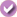Yes, I Did This Project! Please log in (or create a free account) to let us know how things went.

## Credits

Sara Agee, Ph.D., Science Buddies
Teisha Rowland, Ph.D., Science Buddies

This science project is based on this exercise:

M&M's® is a registered trademark of Mars, Inc.

General citation information is provided here. Be sure to check the formatting, including capitalization, for the method you are using and update your citation, as needed.

### MLA Style

Science Buddies Staff. "M&M Math." Science Buddies, 28 July 2017, https://www.sciencebuddies.org/science-fair-projects/project-ideas/Math_p021/pure-mathematics/mms-math?class=AQUwtoaSmYuFfVSIkwtl8nywNbzAix0jiEeuT_KUy3Qs-iBUYwZys13_F6SvZsIniEfV9uEL98c0zkTj9RccMNVn. Accessed 20 Sep. 2019.

### APA Style

Science Buddies Staff. (2017, July 28). M&M Math. Retrieved from https://www.sciencebuddies.org/science-fair-projects/project-ideas/Math_p021/pure-mathematics/mms-math?class=AQUwtoaSmYuFfVSIkwtl8nywNbzAix0jiEeuT_KUy3Qs-iBUYwZys13_F6SvZsIniEfV9uEL98c0zkTj9RccMNVn

Last edit date: 2017-07-28

## Introduction

"Life is like a box of chocolates—you never know what you're going to get" (Forrest Gump in Forrest Gump, 1994).

The "Forrest Gump Chaos Theory" states that in a population of chocolate product (i.e., a box of chocolates) the frequency of a specific outcome (i.e., picking a caramel filled, creme filled, nougat filled, etc., chocolate candy) is unknown and hence unpredictable. I beg to differ! An alternative hypothesis is that the frequency of these outcomes can be modeled and predicted by using simple statistical analysis (i.e., math). This may sound complicated, but this science project will make this hypothesis easy to understand and test for yourself.

Sometimes statistics can be unpredictable, meaning that they do not have any kind of pattern. In other words, the numerical information they are based on appears to be random. However, many statistics do reveal patterns and can be used to make models, form hypotheses, and make predictions about certain things. For example, if you figured out the average height of the students in your class, that statistic could help you predict the heights of students in another class at your school that is in the same grade as yours.

What if you wanted to know how many students in your class are over four feet tall? How could you use statistics to investigate and explain this? You could count how many students are over four feet tall in your class and compare this to the total class size. In statistics, how often a certain event happens, such as a student being over four feet tall, is referred to as the frequency of that event. As shown in this example, frequency can be measured by counting the numbers of different types within a group (like the numbers of each different type of chocolate in a box of chocolates). In this mathematics science project, you will determine the frequency of different colored M&M's in a package of M&M candies (M&M's are much cheaper than a box of chocolates). The six colors of M&M's candies are shown in Figure 1.Figure 1. In this science project you will investigate the frequency of each M&M color in a package of M&M's. In this image, the frequency of each color is the same (there are three of each color). What do you think the frequencies will be like in an actual package of M&M's?

What do you think the most common M&M color will be in a package of M&M's? Will your statistics show a pattern? How will different types of graphs help reveal the patterns and allow you to make different comparisons? Is the "Forrest Gump Chaos Theory" credible, or can you predict what M&M color(s) you are most likely to pick?

## Terms and Concepts

• Hypothesis
• Statistics
• Numerical information
• Population
• Prediction
• Event
• Frequency

### Questions

• What is statistics? How is it used to explain something?
• How is frequency related to an event?
• Which color do you think will be the most common one in a package of M&M's?

## Bibliography

Do further research by visiting the following websites, which give information about statistics, frequency, and different areas of math to help review math skills:

You can print out graph paper for this science project from this website:

For help creating graphs, try this website:

This science project is based on this exercise:

## News Feed on This Topic, ,
Note: A computerized matching algorithm suggests the above articles. It's not as smart as you are, and it may occasionally give humorous, ridiculous, or even annoying results! Learn more about the News Feed

## Materials and Equipment

• Standard-size packages of plain M&M's (at least 5, each holding 47.9 g)
• Optional: Plain graph paper, polar graph paper, and colored pencils or markers. Both types of graph paper are freely available at Incompetech.
• Lab notebook

## Remember Your Display Board Supplies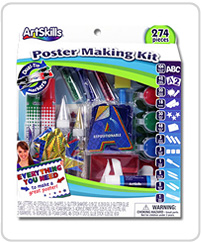Poster Making Kit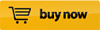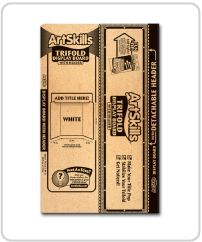ArtSkills Trifold with Header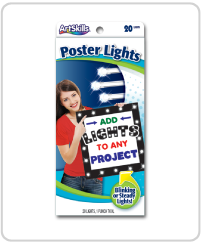Poster Lights## Experimental Procedure

1. In this experiment you will be counting the numbers of different colors of M&M's to calculate the frequencies of the different colors. To do this, first make a data table like Table 1 below in your lab notebook to keep track of your data.

 Candy Color Package Total Average Percentage 1 2 3 4 5 Brown Blue Green Yellow Orange Red Whole Bag
Table 1. In your lab notebook, make a data table like this one to record the number of M&M's of each color in each package.
1. Open the first package of M&M's. Count the number of M&M's of each color and write the numbers in your data table. Do not eat the M&M's before you count them!
2. Repeat step 2 for each package of M&M's.
1. You should sample at least five packages, but you can do as many as your parents will let you open. The more samples you take, the better your data will be. However, too many samples and you might get a tummy ache!
3. Calculate the total number of M&M's in each package.
1. Do this by adding down each column.
2. For each package, write the answer in the "Whole Bag" box in the bottom row of your data table.
4. For each candy color, calculate the total number in all of the packages combined. Also calculate the total number of M&M's in all of the packages combined.
1. Do this by adding across each row.
2. Write the answers in the "Total" column of your data table.
5. Calculate the average number of each candy color per package. Also calculate the average number of candies per package.
1. Do this by dividing the total numbers you calculated in step 5 by the number of packages (which should be 5 or more).
2. Write the answers in the "Average" column in your data table.
6. Calculate the percentage of each colored M&M per package using the average data.
1. Do this calculation by dividing the average number of each color (calculated in step 6) by the average number of M&M's in the whole bag and then multiplying your answer by 100.
1. For example, if there are an average of 5 red M&M's in each bag and an average of 50 M&M's in a whole bag, you will divide 5 by 50 (which equals 0.10) and then multiply by 100 (which gives 10%).
2. Write the answers in the "Percentage" column in your data table.
3. Note: If you do this same calculation with your whole bag data, you should get 100%.
7. Make a bar graph using the data from the "Average" column of your data table. It will tell you the frequency of each color of M&M in the package. It is useful for comparing the frequencies of each individual color to each of the other individual colors.
1. You can make this graph by hand or use a website like Create a Graph to make the graph on the computer and print it.
2. To make the bar graph, label the y-axis (the vertical axis going up and down) with a scale representing the average numbers of M&M's. The smallest number of the scale (minimum) will be zero and the largest number of the scale (maximum) will be set just above the largest average number of M&M's in any color.
1. For example, if the largest average number of M&M's counted was 25 brown M&M's, then make the scale on the y-axis go up to 30.
3. On the x-axis of the bar graph (the horizontal axis going left and right), make a bar for each color. Make the bar go up to the number on the y-axis that corresponds to the average number of M&M's counted for that color.
1. You may want to order the bars on your bar graph from smallest to largest.
2. Label each bar with the correct color (and, if possible, color in the bar with the matching color too).
4. Label the bar graph with a title, such as "Average Number of Each M&M Color in a Package."
8. Looking at your data in the bar graph, try to answer the following questions:
1. In the average package of M&M's, which color are most of the M&M's (highest frequency)?
2. Which color is the rarest (lowest frequency)?
3. Do any of the colors have the same frequencies?
4. Do you see any other trends in your data in the bar graph?
9. Now you are ready to make your second graph, the pie graph, using the data from the "Percentage" column of your data table. It will tell you which portion of the whole bag is of each color. It is useful for comparing the relative proportion of each individual color to the whole population.
1. You can make this graph by hand or use a website like Create a Graph to make the graph on the computer and print it.
2. Using polar graph paper, make a pie graph where each slice colored in is equal to the percentage of one of the colors.
1. For example, if blue M&M's are 15% of a bag, and if your polar graph paper has a slice marked for every 5 units, then color in three of these slices for the blue M&M's.
2. Label each slice with the correct color (and, if possible, use a matching color for each section). You can even write the percent data inside each slice.
3. Make a title for your graph, such as "Percentage of Each M&M Color in a Package."
10. Looking at your data in the pie graph, try to answer the following questions:
1. What is the percentage of each color of M&M in the package?
2. Does any color make up more than half of a package? What about more than a third of the package?
11. Looking at your data table and graphs, do you think you can predict what color(s) M&M you are most likely to pick from a package? If so, what color(s) is it?

## If you like this project, you might enjoy exploring these related careers:

### Statistician

Statisticians use the power of math and probability theory to answer questions that affect the lives of millions of people. They tell educators which teaching method works best, tell policy-makers what levels of pesticides are acceptable in fresh fruit, tell doctors which treatment works best, and tell builders which type of paint is the most durable. They are employed in virtually every type of industry imaginable, from engineering, manufacturing, and medicine to animal science, food production, transportation, and education. Everybody needs a statistician! Read more

### Actuary

Life is full of risks to both people and property. Actuaries predict the chances that future negative events will occur in a person's life, and then think of ways to reduce those chances, or reduce the impact of those negative events. Actuaries help bring peace of mind to both families and to businesses. Read more

### Math Teacher

Math teachers love mathematics and understand it well, but much more than that, they enjoy sharing their enthusiasm for the language of numbers with students. They use a variety of tools and techniques to help students grasp abstract concepts and show them that math describes the world around them. By helping students conquer fears and anxieties about math, teachers can open up many science and technology career possibilities for students. Teachers make a difference that lasts a lifetime! Read more

## Variations

• You could try this science project with other types of M&M candies, such as peanut or pretzel M&M candies instead of plain M&M candies. Is there much variation in the colors that different types of M&M candies have?
• To really test the "Forrest Gump Chaos Theory" you could invest a bit more cash, and talk your parents into springing for 10 boxes of chocolates. For those investigators on a tight budget, you can do a similar statistical experiment with almost any other product that comes packaged with a mixture of colors, shapes, sizes or types. Here are more ideas: Skittles, jellybeans, a bag of chips, marbles, Pokemon cards, etc. Can you accurately predict what you are most likely to pick from one of these packages?
• You can use the same kind of statistical analysis for more advanced comparisons: the number of different languages spoken by students in your school, the different types of pets owned by different people, car ownership of families at your school, number of seeds sprouting per seed packet, how tall people are at your school, how many girls and boys in each class or grade, etc. Figure out how best to use statistics to describe different parts of the world around you.
• Another more advanced way to look at frequencies is to look at a distribution of frequencies to see how much variation there is in your data set. You can do this by making a different kind of graph, called a histogram, for each color from each package. Do some background research on histograms to figure out how to make one. On the x-axis (horizontal axis) you can separate the colors from each package by their frequency. For example, you could divide the x-axis into 0 to 5 candies, 6 to 11 candies, 12 to 17 candies, etc. On the y-axis (vertical axis) keep track of the number of packages used. For example, if you are making a histogram on green M&M's and one package has 3 green M&M's, two packages have 10 green M&M's, a fourth package has 8 green M&M's, and a fifth package has 4 green M&M's, on your histogram you would put two packages in the 0 to 5 candies category, and three packages in the 6 to 11 candies category. For each color, what is the most common amount of candies to find in a package?

## Share your story with Science Buddies!Yes, I Did This Project! Please log in (or create a free account) to let us know how things went.

## Ask an Expert

The Ask an Expert Forum is intended to be a place where students can go to find answers to science questions that they have been unable to find using other resources. If you have specific questions about your science fair project or science fair, our team of volunteer scientists can help. Our Experts won't do the work for you, but they will make suggestions, offer guidance, and help you troubleshoot.